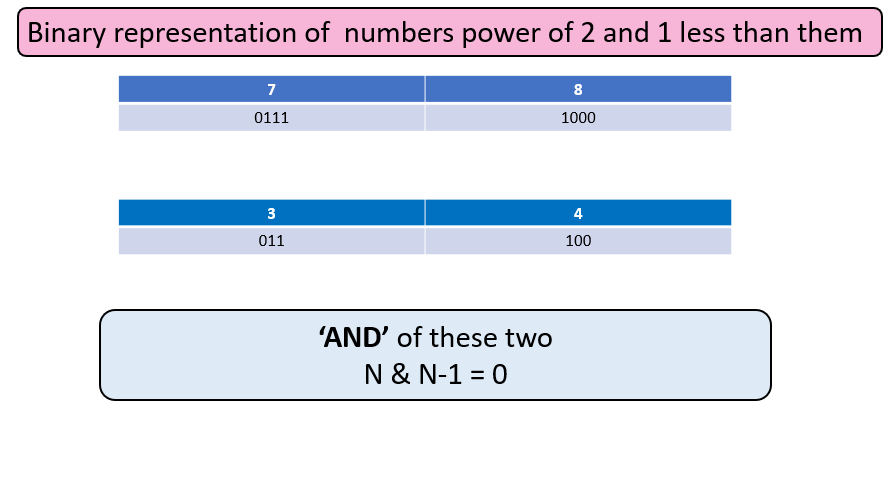# Power of Two

Problem Statement: Given a positive integer, we have to check whether it is a power of two or not.

Examples:

```Example 1:
Input: N = 4
Output: true
Explanation: 2*2 = 4

Example 2:
Input: 9
Output: false
Explanation: It cannot be represented in powers of 2
```

### Solution

Disclaimer: Don’t jump directly to the solution, try it out yourself first.

### Solution 1:

Approach 1 is very simple where we will take the log to the base 2 and check if the result is an integer or not. If it is, we will print “YES” else we will print no.

For Eg,

```N = 4
log2(4) = 2
log2(9) = 3.169 (approx)```

Code:

## C++ Code

``````#include <bits/stdc++.h>
using namespace std;
// Function to check power of 2
bool checkpowerof2(int n)
{
return ceil(log2(n)) == floor(log2(n));
}
int main()
{
int n=64;
if(checkpowerof2(n)==1)
cout << "true" << endl;
else
cout<<"false"<<endl;
return 0;
}
``````

Output: true

Time Complexity: O(1)

Space Complexity: O(1)

## Java Code

``````import java.util.*;
public class Main {
public static boolean checkpowerof2(int n) {
return (int)(Math.ceil(Math.log(n) / Math.log(2))) == (int)
(Math.floor(Math.floor(Math.log(n) / Math.log(2))));
}
public static void main(String args[]) {
int n = 64;
System.out.println(checkpowerof2(n));
}
}
``````

Output: true

Time Complexity: O(1)

Space Complexity: O(1)

### Solution 2:

Approach 2 is based on dividing the number by 2 again and again until we get 1.

For Eg –

```n = 7

7/2 = 3 ( we will return false here
only since the number is
not divisible by 2 )

n = 4
4/2 = 2
2/2 = 1 (1 is found, also at every
step number is divisible so
return true )```

Code:

## C++ Code

``````#include <bits/stdc++.h>
using namespace std;
// Function to check power of 2
bool checkpowerof2(int n)
{
while(n!=1)
{
if(n%2!=0) return false;
n=n/2;
}
return true;
}
int main()
{
int n=64;
if(checkpowerof2(n)==true)
cout << "true" << endl;
else
cout<<"false"<<endl;
return 0;
}
``````

Output: true

Time Complexity: O(log2(n))

Space Complexity: O(1)

## Java Code

``````import java.util.*;
public class Main {
public static boolean checkpowerof2(int n) {
while(n!=1)
{
if(n%2!=0) return false;
n=n/2;
}
return true;
}
public static void main(String args[]) {
int n = 64;
System.out.println(checkpowerof2(n));
}
}
``````

Output: true

Time Complexity: O(log2(n))

Space Complexity: O(1)

### Solution 3:

This approach is based on the bit manipulation. Here we will check if the number has only 1 set bit.

For Eg –

```___________________________
For 8, binary representation : 1000
It means it is a power of 2
___________________________
For 3, binary representation - 11
It is not a power of 2
___________________________```

Let’s observe something !!!From above, it can be observed that if i take “And” of the number and its 1 less  will give me zero if and only if it is the power of 2

Code:

## C++ Code

``````#include <bits/stdc++.h>
using namespace std;
// Function to check power of 2
bool checkpowerof2(int n)
{
return !(n & n-1);
}
int main()
{
int n=64;
if(checkpowerof2(n)==true)
cout << "true" << endl;
else
cout<<"false"<<endl;
return 0;
}
``````

Output: true

Time Complexity: O(1)

Space Complexity: O(1)

## Java Code

``````import java.util.*;
public class Main {
public static boolean checkpowerof2(int n) {
return (n & n-1)==0;
}
public static void main(String args[]) {
int n = 64;
System.out.println(checkpowerof2(n));
}
}
``````

Output: true

Time Complexity: O(1)

Space Complexity: O(1)

Special thanks to Gurmeet Singh for contributing to this article on takeUforward. If you also wish to share your knowledge with the takeUforward fam, please check out this article